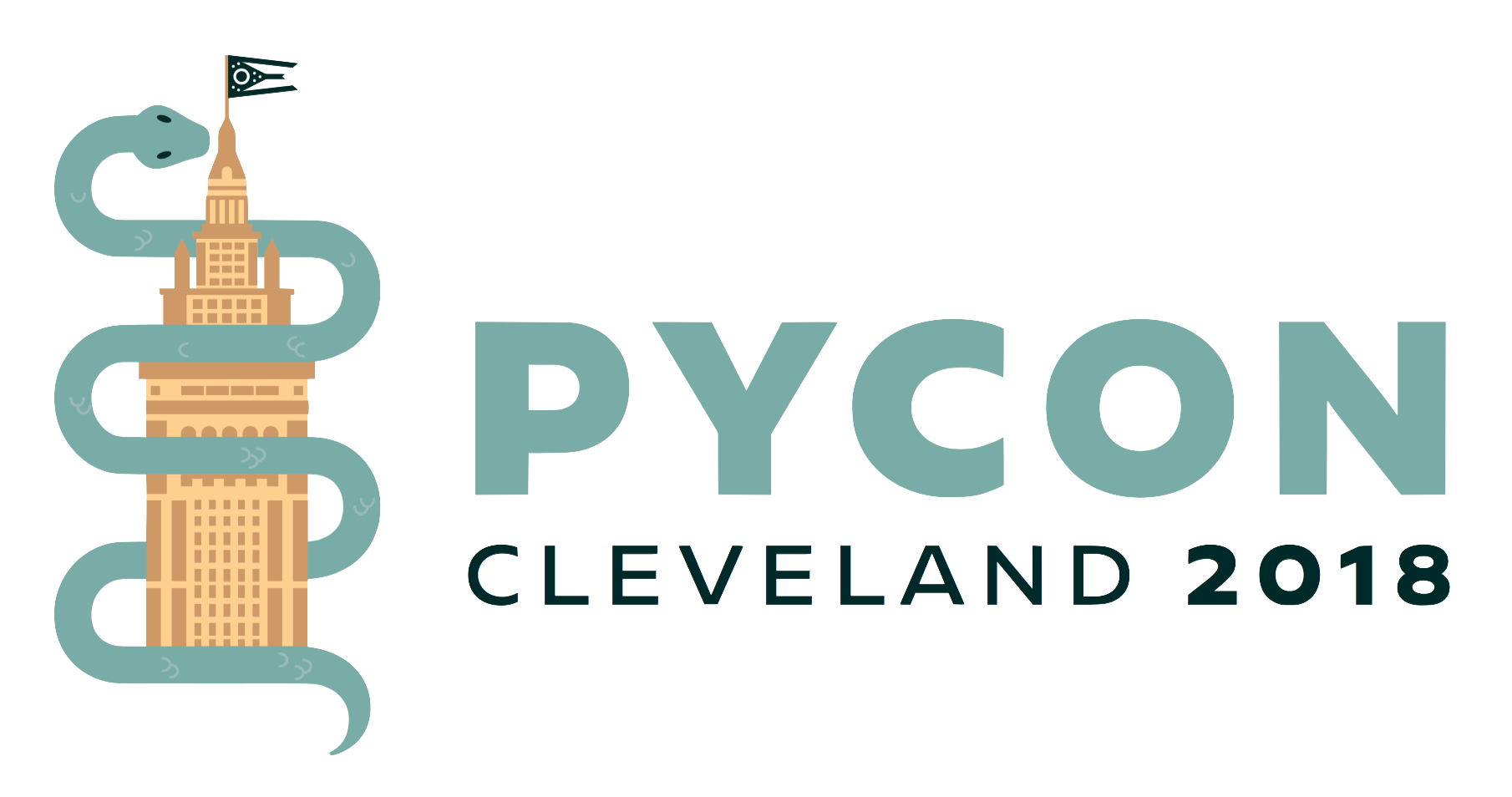## Bayesian Non-parametric Models for Data Science using PyMC3

### Description

Nowadays, there are many ways of building data science models using Python, including statistical and machine learning methods. I will introduce probabilistic models, which use Bayesian statistical methods to quantify all aspects of uncertainty relevant to your problem, and provide inferences in simple, interpretable terms using probabilities. A particularly flexible form of probabilistic models uses Bayesian *non-parametric* methods, which allow models to vary in complexity depending on how much data are available. In doing so, they avoid the over-fitting that is common in machine learning and statistical modeling. I will demonstrate the basics of Bayesian non-parametric modeling in Python, using the PyMC3 package. Specifically, I will introduce two common types, Gaussian processes and Dirichlet processes, and show how they can be applied easily to real-world problems using two examples.